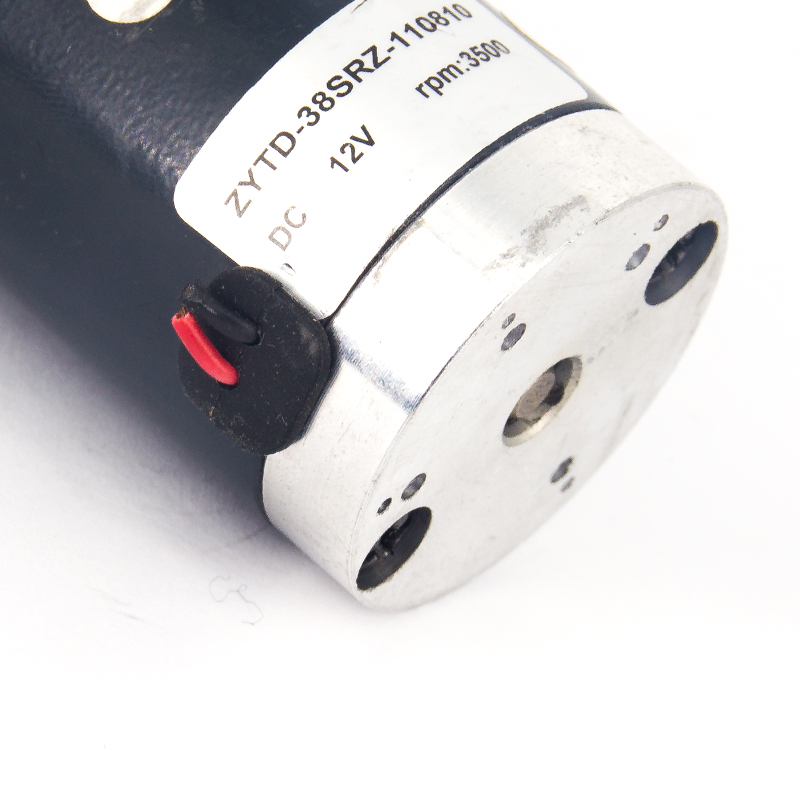# The relationship between torque load, speed, and motor current of brushed DC motor

This article will take the brushed DC motor as an example to discuss the relationship between the torque load of the brushed DC motor, the speed of the brushed DC motor, and the motor current of the brushed DC motor.Relationship between torque load, speed, and motor current

The relationship between the torque load, speed, and motor current of the brushed DC motor is: when the torque load is zero, the motor rotates at the maximum speed, and when the torque load is applied, the speed will decrease inversely proportional to the magnitude of the torque load; When the torque load is zero, the motor current is minimum, and the motor current increases proportionally to the torque load, and when the speed is zero, the motor current is maximum.

When the load torque to the motor is zero, the speed is maximum; when the load torque gradually increases, the speed decreases proportionally to the load torque, and the speed becomes zero at a certain load torque. The torque at this point is the maximum load torque. The motor current increases in inverse proportion to the load torque, and the load torque is maximum when the speed becomes zero.

In an actual motor, even in a no-load state, a slight motor load torque may be applied due to the friction of the motor itself, so the current does not become zero even at the maximum speed of the motor. If under ideal conditions, the motor load torque can become zero, then the ideal torque will be presented. When the power supply voltage increases, the maximum speed and maximum load torque increase, and the maximum current value also increases.

### Contacts

86-18657763332 sales@zhengkemotor.com NO.221,Shahong Road, Bantang Industry Zone,Beibaixiang,Yueqing,Zhejiang-325603,China